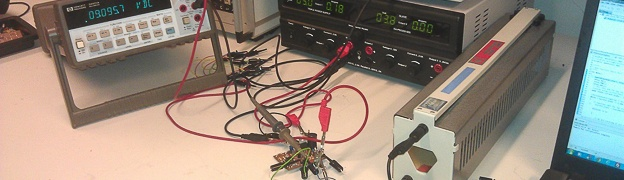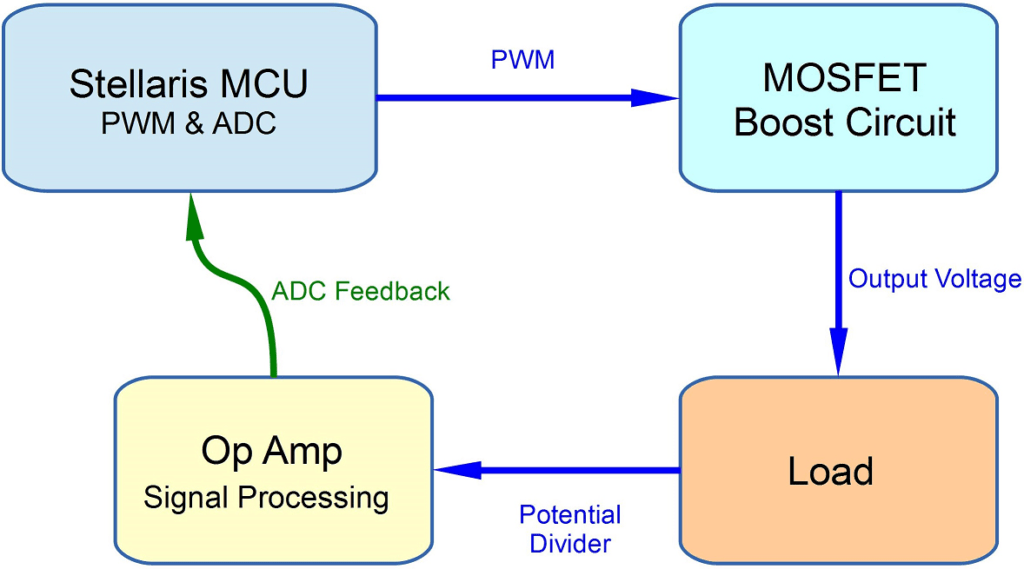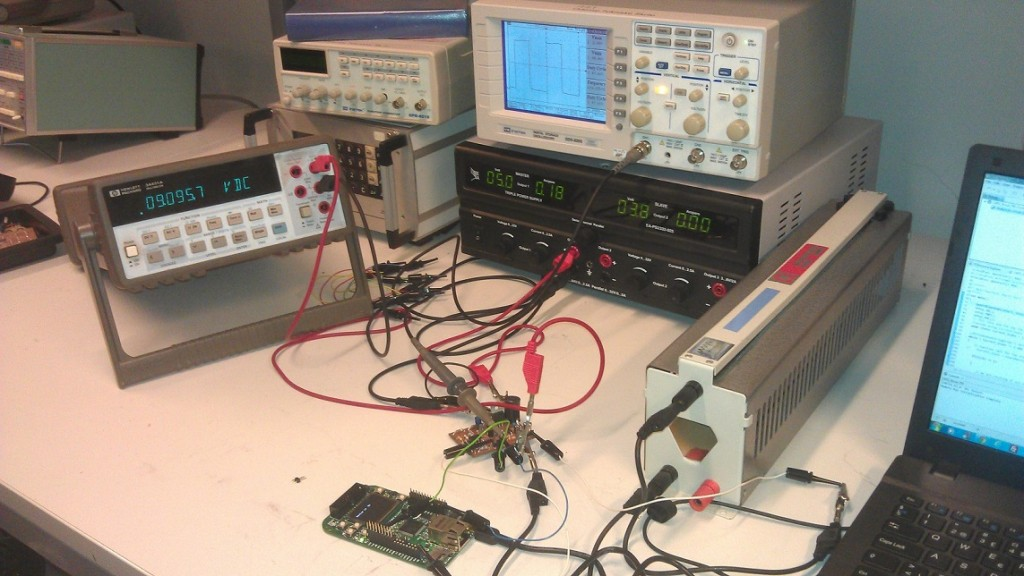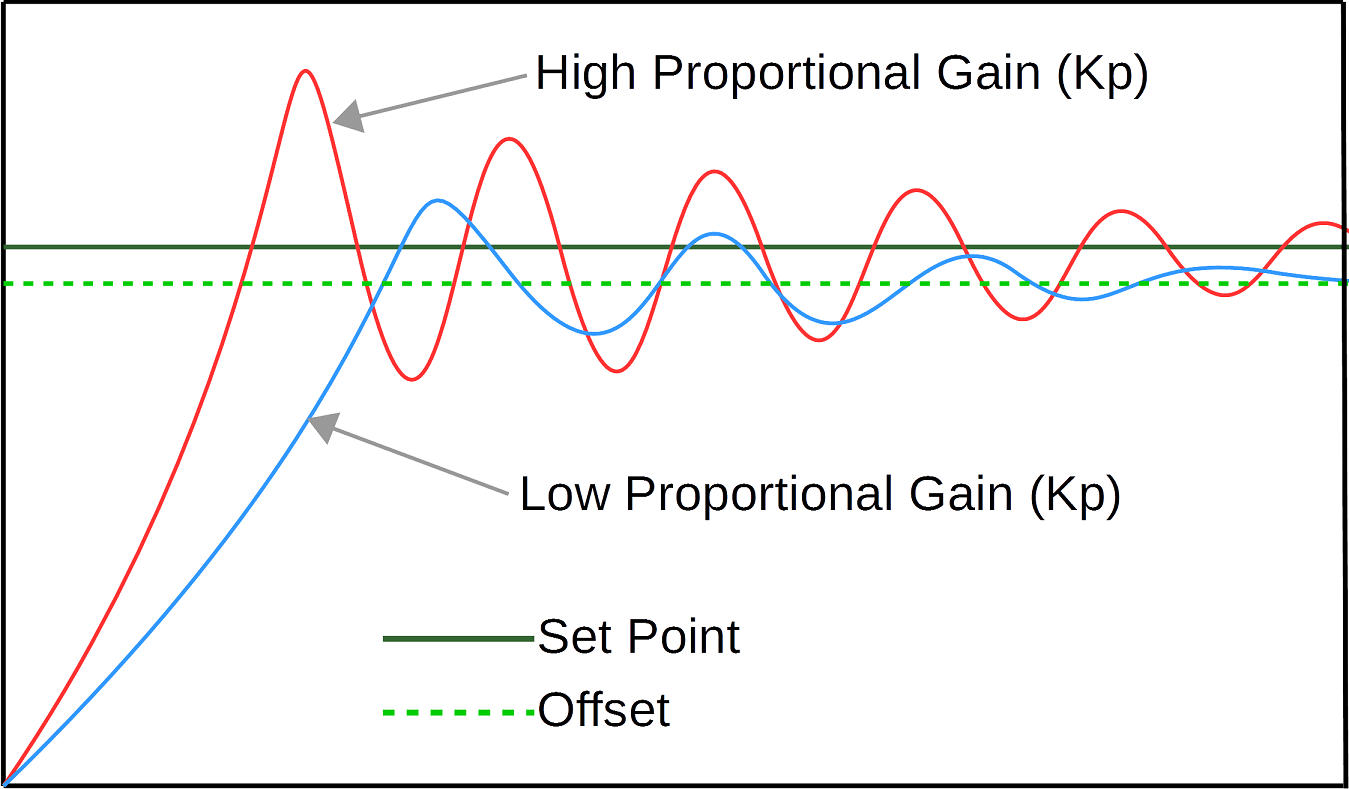# PID Tutorial C code Example Pt/2

In this PID tutorial C code example Pt/2, the basic concept of a Boost PID controller will be explored.  All the PID controller C code is stepped through, as well as some basic concepts for testing and tuning the controller.  The final C code example can be downloaded via the link at the end of the article.

## Hardware Overview

The image below shows a rough blocked out diagram of the boost circuit and feedback loop.As can be seen it’s a fairly simple design, with the output voltage being monitored and fed back to the LM3S6965.

A schematic for the boost converter can be seen below, this circuit was originally made to test a variable voltage boost converter.  The original design would use a 5V input which would then be boosted to either 9V, 12V or 15V, this was controlled via a state machine running on the Stellaris LM3S6965.The drive circuit for the mosfet uses 2 transistors in a totem pole configuration, and a 3rd transistor is to ensure a small current is sourced from the LM3S6965.  The main boost circuit itself consists of L1, Q1, D1 and C3.  The sampled output voltage via the potential divider is first passed through an operational amplifier U1A, configured as a non inverting amplifier which provides a gain of 2.2.  The operational amplifier U1B acts as a unity gain buffer, it’s input is connected to 3 resistors R7, R8 and R9.  These 3 resistors provide the offset voltage of 770mV and U1B ensures there is a high impedance input.  The output of U1B feeds the offset voltage to R4 and then to the negative input on U1A (effectively offsetting ground).  The boost converter was originally set-up to measure voltages between 4V and 19V.  So the operational amplifier arrangement with offset, ensures the sampled voltage range uses almost the full range of the ADC.  For the PID testing the output voltage will be regulated to 9V, which is equal to 353 on the ADC which is also the set point value.

## Software

The software used was written after reading various sources on PID.  It has been designed so it is easy to understand, as it uses local and global variables.  A more efficient way would be to use a typedef structure or typedef struct in C.

So firstly we have our constants and global variables.

```/* constants for PID */
const float Kp = 0.01;
const float Ki = 0.01;
const float Kd = 0.001;
const int Set_Point = 353;

/* Global Variables for PID */
float d_Temp = 0;
float i_Temp = 0;
float PWM_Temp = 166;```

In the first part of this tutorial the proportional (Kp), integral (Ki) and derivative (Kd) constant values were mentioned.  These are the values which provide the tuning for the PID control system.  The next constant is the set point, this was observed as the read ADC value, when 9V was present on the output.  The 9V was measured and adjusted using a multimeter with a fixed load on the output, the duty cycle had a fixed value which was then increased until 9 volts was reached.  The first 2 global variables are used to store the accumulated and historic error values required for integration and differentiation.  The global variable PWM_Temp is initially given a value, then this is used to store the old duty cycle value, which is then used in the new duty cycle calculation.

```void PWM_Duty_Change()
{
//Local variables for PID
float iMax = 100;
float iMin = -100;
float Err_Value;
float P_Term;
float I_Term;
float D_Term;value
float PWM_Duty;```

The next set of variables are all local to the PID function called PWM_Duty_Change(), all the code that follows will be inside this function, until the closing brace of the function is stated and mentioned.  Additionally the entire code which was used on the LM3S6965 can be downloaded (bottom of the page), so it can be seen how and when the function was called.

The first 2 local variables are called iMax and iMin, these are used to prevent integral windup.  More will explained on integral windup further in the tutorial, but for now we will just run through the variables.  The next 4 variables have already briefly been touched upon in part 1 of this tutorial, these are Err_Value, P_Term, I_Term and D_Term.  Then we are left with new_ADC_value which is simply the latest sampled value form the ADC, and finally PWM_Duty which will be the new PWM duty cycle value passed to the timer module.

`new_ADC_value = read_ADC();`

The read ADC code does not need much explanation, other than a function was created to read the ADC and return the value as an integer.  If you are using this code on another microcontroller, you simply need to construct an ADC read function, then insert instead of the read_ADC().

`err_value = (Set_Point - new_ADC_value);`

Going back to part 1 of this tutorial it was shown that the error value can be obtained, by simply taking the newest error value away from the set point, that’s all this line performs.

`P_Term = Kp * Err_Value;`

To obtain the proportional term the Kp constant is multiplied by the error value.

`i_Temp += Err_value;`

The accumulated error value is used in integration to calculate the average error, so first the current error value must be added to the accumulated value, and that’s what this line performs.

```if (i_Temp > iMax)
{i_Temp = iMax;}
else if (i_Temp < iMin)
{i_Temp = iMin;}```

The if and else if statements are used to cap the accumulated integral term, by simply setting upper and lower thresholds.

`I_Term = Ki * i_Temp;`

The integral term is then calculated by multiplying the Ki constant with the accumulated integral value.

```D_Term = Kd * (d_Temp - Err_Value);
d_Temp = Err_Value;```

The first line of this code snippet calculates the derivative term.  To understand how the code works the second line needs to be run through quickly, this basically assigns the newest ADC value to the d_temp global variable.  So the d_Temp variable effectively stores the historic error value, therefore allowing the derivative to be calculated.  Now looking at the first line again, the newest ADC value is taken away from the historic value, the difference between the 2 would allow the rate of change to be calculated (assuming the time between the readings is a known quantity). Then this value is multiplied by the derivative constant and assigned to the derivative term.

`PWM_Duty = PWM_Temp - (P_Term + I_Term + D_Term);`

Now that we have all the PID terms calculated, they can be used to change the PWM duty cycle.  This next piece of code adds all the PID terms together, then they are subtracted from the PWM_Temp variable (PWM_Temp usage is shown shortly as it comes towards the end of the function).  This value is then assigned to the PWM_Duty variable.  The way the PID terms are used here is specific to this program, firstly the sum of the PID terms is subtracted, this is due to the final electronic circuit used.  What’s important here is the PID terms are summed, then they need to be used in such away as to construct a valid PWM duty cycle or other chosen control method.

```if (PWM_Duty > 300)
{PWM_Duty = 300;}
else if (PWM_Duty < 30)
{PWM_Duty = 30;}```

The if and else if statements are used to prevent the duty cycle from going too high or too low, in this case this simply prevents the output voltage from going too high or too low.  If say you were using a half bridge driver integrated circuit, these usually state a maximum duty cycle of say 99%, if this is exceeded the voltage doubler cannot function correctly, so having limits can be a good protection system.

`adjust_PWM(PWM_Duty);`

This line is again limited to this specific application, it simply calls the function adjust_PWM(), which has the new PID calculated duty cycle value, as a parameter.

```PWM_Temp = PWM_Duty;
}```

The final statement in the function before the closing brace, assigns the current PWM duty cycle value to the PWM_Temp global variable.

Integral windup can occur if the integral term accumulates a significant error, during the rise time to the set point.  This would cause the output to overshoot the set point, with potentially disastrous results, it can also cause additional lag in the system.  The integral windup prevention used in this example, places a cap on the maximum and minimum value the integrated error can be.  This effectively sets a safe working value and is considered good working practice in any PID system.  The values used for integral windup as with the PID constant gain values are specific to this system, these would need to be determined and tuned to your system.

## Step Response

The intention was to also explore the well documented Ziegler-Nichols approach, however time constraints for the project did not allow this method to be fully explored.  Some basic concepts of how to carry out a step response test will be shown, as I am sure it will prove useful.

The systems step response shows how the system responds to a sudden change, electronic circuits switch quickly, so an oscilloscope was the best piece of equipment for the job.  The boost converter was set up with a fixed load, a fixed PWM duty cycle and no feedback , therefore in an open loop configuration.  Then some additional test code was added, initiating these 2 extra functions when a button was pressed on the LM3S6965 development board :

1. A free GPIO pin would be taken from low to high
2. The PWM duty cycle would decrease by 10% effectively increasing the output voltage

The free GPIO pin would be connected to channel 2 on the oscilloscope, and in this way can be used as a trigger to start a capture event.  Channel 1 on the oscilloscope would be connected to the output of the boost converter, thereby capturing the step response as the output voltage rises.  The image below is a photograph of the captured step response on the oscilloscope.The GPIO pin was polled before the PWM duty cycle was updated, however this happens so quickly, it is difficult to distinguish this.  The lower square wave type step is the GPIO pin on channel 2, and the top curved step response is the output from the boost converter.  It can be seen to overshoot it’s settling level at the beginning then oscillate for a time before settling down, this oscilloscopes accuracy wasn’t the best, using a longer time base did not show any extra detail on the oscillations.

If the step response was of a first order, a calculation based on the reaction curve could be performed.  However the step response from the boost circuit is of a second order, and has an under-damped nature.  In order to explore this calculation further, requires another tutorial altogether, however further reading on this can be found here.

The step response of a similar system under the control of a PID controller can also be viewed.  This can be achieved by using a similar set up, the external pin is used again to trigger an oscilloscope capture.  That same GPIO pin is also used to switch a mosfet, which places an additional load in parallel with the output of the boost converter.  The PID algorithm will then regulate the output voltage to ensure it meets the set point with the new load, and the step response can then be captured.

## Testing and Tuning

The system was set up and a basic tuning approach was used, the image below shows the system on the test bench.The image shows the following pieces of equipment:

• Hewlett Packard multimeter connected to the output, monitoring the output voltage
• Bench power supply, supplying 5V to the circuit
• Oscilloscope, used to monitor the PWM duty cycle
• 0-100Ω bench potentiometer
• Boost converter PCB, LM3S6965 development board and a laptop to tweak the code

With everything connected the PID algorithm was set up, but with only the proportional part in operation.  The set point was set to 353 as mentioned previously, which produced close to 9V give or take 10-15mV.  The load was fixed and set to 100Ω.  Initially the proportional gain constant Kp was set very low around 0.001, this was too low so it was increased over a few steps until 0.01.  At this point the output voltage was observed to be approximately 7.5V, and the duty cycle waveform was observed to have a small amount of jitter, as the duty cycle fluctuated +/- 0.5%.  The Kp value was again increased, until the output voltage read approximately 8V, the duty cycle was again observed and found to be fluctuating by a greater amount, by approximately +/-5%.  The increase in jitter on the PWM duty cycle can be equated to greater oscillations on the output voltage, the multimeter was not suitable for observing these.  It would have been possible to see them on the oscilloscope but this probably would have involved a stop capture then restart method, therefore to make the process quicker the PWM duty cycle was considered ‘good enough’.

At this point the Kp value was backed off, to the previous level of 0.01 so the output voltage was at 7.5V.  This point can be considered close to the offset level mentioned in part 1 of this tutorial, and for ease of reading the image showing the offset level can again be seen below. This image also illustrates again how too larger Kp value can introduce instability in the output.Now with this level reached, the integral part of the algorithm was introduced.  All the hardware was untouched, just a simple modification to the algorithm was made.  The initial value chosen for Ki was 0.01.  This was then loaded on to the LM3S6965 and the output voltage on the multimeter was immediately observed to read 9.02V, +/-10mV.  The oscilloscope was also observed to have minor jitter as before of only a few half percent.  The integral value was not tweaked any further, but in most cases tuning the Kp and Ki values would take a greater time and under a wide range of conditions.

With the PI controller working on a basic level, the next test was to see how it performed under different load conditions.  First the bench potentiometer was left at 100Ω and the input current and output voltage were noted, the wiper was then moved to the middle (approximately 50Ω) and again the output voltage and input current were noted.  At 100Ω the output voltage was 9.02V with a current of 180mA, at 50Ω the output voltage was 8.99V with a current of 500mA, both output voltages still showed minor voltage variations in the +/-10mV range.

The next step was to move the potentiometer wiper rapidly between the 100Ω and 50Ω position, then observe the output voltage and duty cycle (not the most scientific test, but goes some way to demonstrating the system).  With this test being performed the duty cycle was observed to rapidly increase and decrease, too quick to observe anything further and a step response would be the best method to use here.  The output voltage was observed to have a minor variations, fluctuating +/-15-20mV

After this test, the derivative constant was introduced and tweaked to find the best settings. The Kd constant was not observed to have a huge affect on the system, unless increased dramatically which produced instability.  This set up is not ideal for testing the derivative term and a more detailed and longer term approach would be best.

The basic test performed seemed to conclude the PI controller was working as per the research carried out.  The system used here updates at a very fast rate, therefore observing the step response at this point would be best practice.  As this system has no critical importance other than to explore PID, it simply wasn’t necessary to tune it to a high degree of accuracy.  I have a number of projects in the works however, which touch on this subject again and I intend to post them when I have time.

## Example Code

The link below contains the zip file with the complete C code.

PID LM3S6965 C code

## 19 thoughts on “PID Tutorial C code Example Pt/2”

1.demelash says:

hey can you send me full code

1.Ant says:

Hi,

The code is definitely downloadable, make sure you turn off all ad-blockers and possibly use Chrome and not Firefox as noticed Firefox does not display adverts so could be the issue.

Regards,
Ant

2.Daniel Pinheiro says:

First of all I would like to congratulate you for your article, I think it is a very nice introduction to PID control! But I suggest that you think about using the period of the loop for calculating the integral and derivative terms.

period = 0.02; //time between 2 cicles of the loop in seconds
integral += integral*period;
D_Term = Kd * (d_Temp – Err_Value)/period.

Without using a time reference, your computational methods will not be integral and derivative, but something else.

Best Regards.

1.Ant says:

Hi Daniel,

Absolutely, this is just a basic introduction, but it would be best to use a timer to trigger the PID algorithm at a particular frequency and then you can incorporate you code snippet.

Regards,

Ant

3.Edmund Soo says:

Hi Ant,

First of all, i would like to said this is an awesome tutorial. 🙂
There is a question I would like to ask which is about the picture of step response in oscilloscope. The channel 1 is output voltage of the boost converter right? But based on the channel 1, the volt/division is only 1V / division, so it shows that it is only 2V you get for your output voltage. I thought your project is boosting from 5V to 9V ?

Sorry that I’m confused on the result shown in oscilloscope, can you please clarify it? 🙂

Sincerely,
Edmund Soo

1.Ant says:

Hi Edward,

Glad this proved useful, I am working on an updated version of the code based on the MSP430 as well.

Fair question, it was awhile now but pretty sure the oscilloscope was connected after the potential divider on the feedback circuit. This would then account for the lower voltage, as the potential divider was setup to ensure the sampled voltage was within the 0-3V range of the ADC.

Regards,
Ant

4.Sivakumar says:

Great Job!!!!!!!

5.andrey says:

Great tutorial, but your code formatting is abysmal.

1.Ant says:

Yep, I have improved my formatting now. Some of the original code uploaded is from when I first started out programming in C.

1.andrey says:

Also a note regarding the algorithm implementation: shouldn’t variables that save values between function calls (I_Temp, D_Temp) be declared as static?

1.Ant says:

In this case I am using Global variables, if the variables were local to the function it would be possible to use static variables.

6.Sergey says:

Hi, thanks for the article, it seems to be one of the easiest to understand articles about PID controllers. One question comes after examining the complete source code, though:

Why in the article the D term is calculated like this:
D_Term = Kd * (d_Temp – new_ADC_value);

and in the attached code example it is calculated like this?
// Calculates Differential value, Kd is multiplied with (d_Temp minus new_ADC_value) and the result is assigned to D_Term
// The new_ADC_value will become the old ADC value on the next function call, this is assigned to d_Temp so it can be used
D_Term = Kd * (d_Temp – Err_Value);
d_Temp = Err_Value;

1.Ant says:

Hi Sergey,

Glad you found it useful, I wrote this as all the tutorials I found did not break it down into a nice easy example. So I thought this will help others and serve as a good introduction to a basic PID algorithm.
When I was writing the tutorial I decided to use variable names that were in-line with the tutorial wording, this way it made more sense and should be easier to understand. The variable names in the downloadable code differ but work in exactly the same way and the calculation is not different, so it should be easy to see the code flow. I was going to change them but simply have not had the time to go through it all.
Best regards,
Ant

1.Sergey says:

Thank you for quick reply. Well, the fact that make me wonder is that in the article and in the code the calculation is NOT the same: in the article you use new_ADC_value to calculate D_Term, and in the code you use Err_Value (which is Set_Point – new_ADC_value), so the difference is not only in the variable names. Which calculation should be considered correct then?

Also, if you don’t mind, please explain, whether it is crucial or not for the PID algorithm to work, to use the PWM_Temp variable (and calculate the PID output previously saved PWM value), or I can just assign the PID output value directly (based only on the P_Term, I_Term and D_Term variables)?

1.Ant says:

Hi Sergey,

Your correct the downloadable code is correct, as the D_Term is the rate of change between the old error value and the current error value, so the tutorial should read (which I have corrected).

D_Term = Kd * (d_Temp – Err_Value);
d_Temp = Err_Value;

I needed away to translate the new PID calculation result, to change the PWM duty cycle value. In this case I used the current PWM duty cycle and copied this to PWM_Temp. This allowed for the PID calculation result to either add or subtract from the current PWM_Temp value, the PID result size would be dependant on the PID variables and the size of the error value. This seemed the simplest solution and worked quite well for the tests that were made.
Unsure how you intend to employ your PID, put simply the result from the PID calculation will produce either a positive or negative value depending on which side of the set-point value the error value is.

Regards,
Ant

7.Dennis says:

Hi Ant

Tnx for the swift reply! I will go through it all and try to use it for my purpose: I have the new Pixy CMUcam5 on my robot and use it in a mode where the X-position of target objects is output with a 0-3.3V analog signal. I want to write my own PI code so I can quickly center the robot w.r.t the middle-point of the target object.

Tnx
Dennis

1.Ant says:

Hi Dennis,

No worries,
This code should be easily portable for your application, and easy to just implement the PI part. Not heard of the Pixy CMUcam5 will have a look into that.

Good luck with the project,
Ant

8.Dennis says:

an awesome tutorial, I am following it right now! Why is the code from the download link different then the example above? Some parameters are commented out like //float P_Error; or //constant float Ki;
And there is no constant float Kd ?

1.Ant says:

Hi Dennis,